Courses

# Origin Of Quantum Mechanics NAT Level – 2

## 10 Questions MCQ Test Modern Physics for IIT JAM | Origin Of Quantum Mechanics NAT Level – 2

Description
This mock test of Origin Of Quantum Mechanics NAT Level – 2 for Physics helps you for every Physics entrance exam. This contains 10 Multiple Choice Questions for Physics Origin Of Quantum Mechanics NAT Level – 2 (mcq) to study with solutions a complete question bank. The solved questions answers in this Origin Of Quantum Mechanics NAT Level – 2 quiz give you a good mix of easy questions and tough questions. Physics students definitely take this Origin Of Quantum Mechanics NAT Level – 2 exercise for a better result in the exam. You can find other Origin Of Quantum Mechanics NAT Level – 2 extra questions, long questions & short questions for Physics on EduRev as well by searching above.
*Answer can only contain numeric values
QUESTION: 1

### The life time of a nucleus in excited state is 10–12s. The uncertainty in the energy and frequency of γ-ray photon emitted by it. (h = 6.63 × 10–34 Js) (in units of 1011 Hz) is a × 1011Hz. Find a.

Solution:

From the energy time uncertainty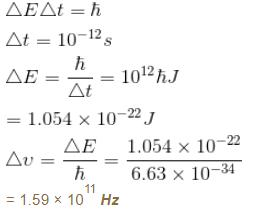The correct answer is: 1.59 x 10^11 Hz

*Answer can only contain numeric values
QUESTION: 2

### The number of photon emitted per second by a 40W source of mono chromatic light of wavelength 6000Å is given by x × 1020. Find the value of x.

Solution:

The correct answer is: 1.206

*Answer can only contain numeric values
QUESTION: 3

### For what wavelength of photon does compton scattering result in a photon whose energy is one half that of the original photon at a scattering angle of 45º? Give the answer as [a × 10–3 Å] . Find the value of a.

Solution: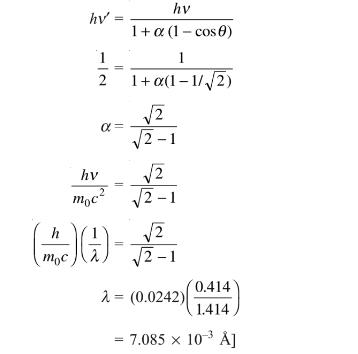The correct answer is: 7.085

*Answer can only contain numeric values
QUESTION: 4

For what value of an electron’s speed will its de-Broglie wavelength be same as the compton wavelength? (in terms of c)

Solution:

de Broglie wavelength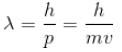Compton wavelength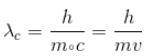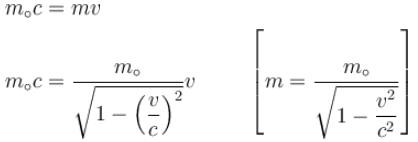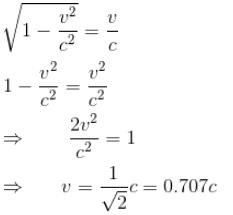The correct answer is: 0.707

*Answer can only contain numeric values
QUESTION: 5

Gamma ray photons of energy 1.02 MeV are scattered from electrons which are initially at rest. Find the angle for symmetric scattering at this energy (in degrees)

Solution:

For symmetric scattering θ = φ
From the relation between  θ & φ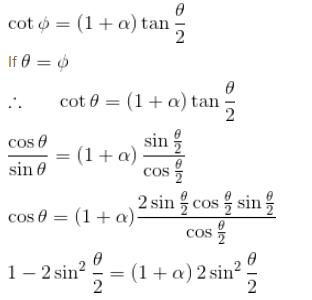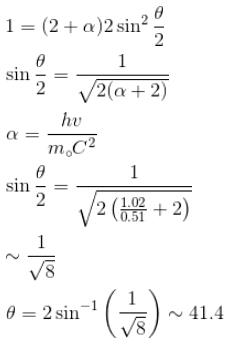The correct answer is: 41.4

*Answer can only contain numeric values
QUESTION: 6

The average life time of an excited atomic state is 10–8s. If the wavelength of the spectral line associated with the transition from this state to the ground state is 6000 Å. What will be the width of this line? (in femtometer)

Solution:

Average life time = Δt = uncertainty in time = 10–8s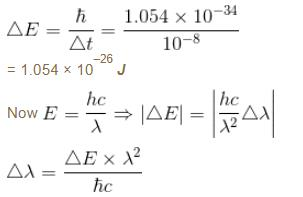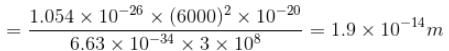width of the line = 1.9 × 10–14 m
The correct answer is: 19

*Answer can only contain numeric values
QUESTION: 7

If the X-ray photon is scattered at angle of 180° and electron recoils with an energy of 4 keV. Then calculate the wavelength of the incident photon (in Å) in angstroms.

Solution:

K.E. of recoiled e is
E = 4 × 103 × 1.6 × 10–19 Joules = 6.4 × 10–16 J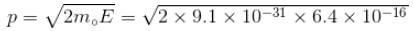= 34.13 × 10–24 kg m/s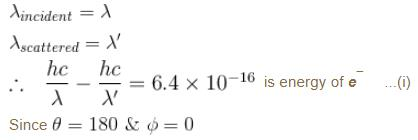From conservation of momentum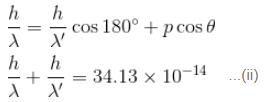solving (i) & (ii)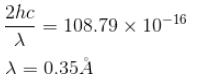The correct answer is: 0.35

*Answer can only contain numeric values
QUESTION: 8

The minimum kinetic energy of an alpha particle that can exist in a nucleus (use uncertainty principle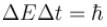)  (Radius of nucleus = 10–14m) (in keV)

Solution:

Radius of nucleus = 10–14 m
∴ Uncertainty in position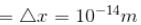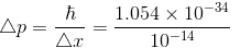= 1.054 × 10–20 kg m/s
Mass of α particle = 4mp = 4 × 1.67 × 10–27 kg
0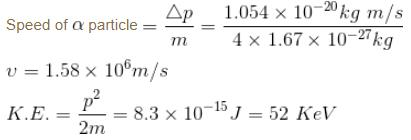The correct answer is: 52

*Answer can only contain numeric values
QUESTION: 9

The intensity of scattered monochromatic beam of X-rays is plotted as a function of wavelength. There are 2 peaks observed. The distance between the 2 peaks is 0.024 Å. Find the angle (in degrees) at which the X-rays are scattered.

Solution: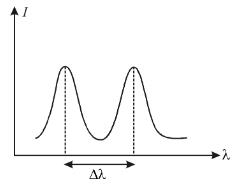Distance between two peaks = Δλ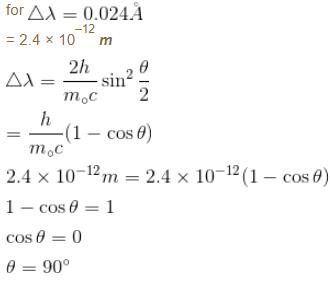The correct answer is: 90

*Answer can only contain numeric values
QUESTION: 10

Calculate the ground state energy of a Helium atom, using the uncertainty principle. (in eV)

Solution:

For a helium atom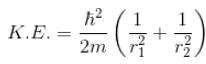Because momentum of electron 1,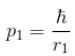and momentum of electron 2,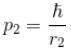p1,  p2 is the spread in momentum corresponding to electron 1 & 2 respectively.

r1r2  is the localization of electron 1 & 2 respectively.

Total energy, E = K.E + Interaction energy of e1 and e2 + Interaction energy between nucleus and electrons

Interaction Energy between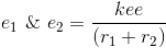Since the separation between r1 and r2 is of the order r1 + r2 and k = 1 in natural system of units.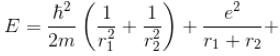Interaction between nucleus and electron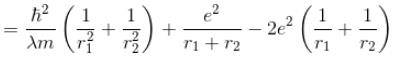For the ground state energy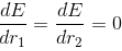since in the ground state, Energy is minimum.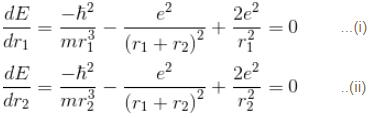solving these two equations, we get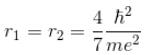substituting r1 and r2 in the expression for E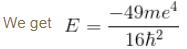Emin = –10.34 eV
Put  m = 9.1 × 10–31 kg
e = 1.6 × 10–19C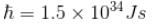The correct answer is: -10.34